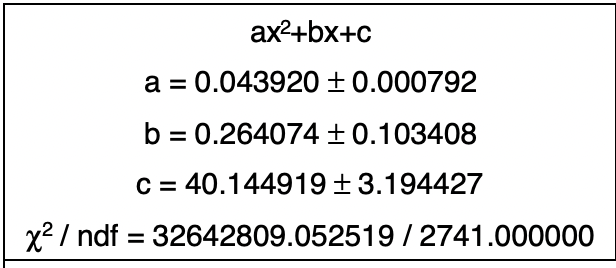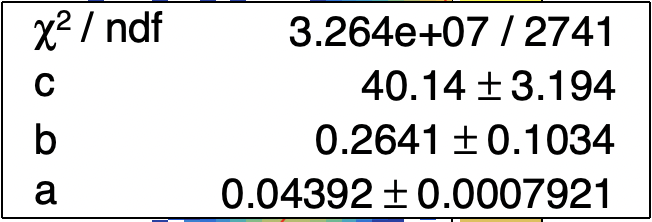# Get chisquare and draw box

I have a macro which fits a TH2F and I want to format the way the parameters are drawn. I would like a box in the top left corner, like what’s drawn, which contains, in this order:

``````ax^2+bx+c
a = Form("a = %f #pm %f",aP,eaP)
b = Form("b = %f #pm %f",bP,ebP)
c = Form("c = %f #pm %f",cP,ecP)
``````

When I tried to draw a `TBox` previously, nothing seemed to appear, probably because I was using NDC coordinates when TBox wants User coordinates. Is there a way of 1. getting the chisquared/NOF of the fit, and 2. of drawing a TBox with the NDC coordinates I want?

``````  TFile *_file0 = TFile::Open("AnalysisOutputFile.root");
gStyle->SetOptStat(0);
gStyle->SetOptFit(100);

gStyle->SetTextFont(42);
gStyle->SetTextSize(0.03);

TLatex *qe = new TLatex(0,0,"ax^{2}+bx+c");

double x1=0.08;
double x2=0.42;
double y1=0.775;
double y2=0.965;

TF1 *fnP = new TF1("fnP","+*x+*x*x");

fnP->SetParName(0,"c");
fnP->SetParName(1,"b");
fnP->SetParName(2,"a");

TH1F *hP = (TH1F*)_file0->Get("hP");

TCanvas *canP = new TCanvas("canP","canP",900,700);
hSwumEnergyToChannelPVeto->Draw("colz");
hSwumEnergyToChannelPVeto->Fit("fnP","","",20,90);
hSwumEnergyToChannelPVeto->SetTitle(";PVetoChannelID;ParticleEnergy");
hSwumEnergyToChannelPVeto->GetYaxis()->SetTitleOffset(1.1);

canP->SetRightMargin(0.13);
canP->SetLeftMargin(0.08);
canP->SetTopMargin(0.0351);
canP->SetBottomMargin(0.075);
canP->SetBorderMode(1);
canP->SetHighLightColor(0);
canP->Modified();
canP->Update();

TPaveStats *sP = (TPaveStats*)hSwumEnergyToChannelPVeto->FindObject("stats");
sP->SetName("P");

TList *lP = sP->GetListOfLines();
double cP = fnP->GetParameter(0);
double bP = fnP->GetParameter(1);
double aP = fnP->GetParameter(2);

double ecP = fnP->GetParError(0);
double ebP = fnP->GetParError(1);
double eaP = fnP->GetParError(2);

sP->SetX1NDC(x1);
sP->SetX2NDC(x2);
sP->SetY1NDC(y1);
sP->SetY2NDC(y2);

TLatex *texaP = new TLatex(0,0,Form("a = %f #pm %f",aP,eaP));
TLatex *texbP = new TLatex(0,0,Form("b = %f #pm %f",bP,ebP));
TLatex *texcP = new TLatex(0,0,Form("c = %f #pm %f",cP,ebP));

hSwumEnergyToChannelPVeto->SetStats(0);

canP->Modified();
canP->Update();
``````

1:

``````fnP->GetChiSquare();
fnP->GetNDF();
``````

2: Not currently (see the TBox documentation). You could do the trick shown here, or use e.g. a TPaveText instead.

1 Like

The TPaveText is a really good solution, but how can I get it to have the same style as a normal stats box would? With number written in standard form and letters left justified while numbers right justified?:

``````  TPaveText *ptP = new TPaveText(x1,y1,x2,y2,"NDC");

ptP->AddText(Form("#chi^{2} / ndf = %f / %f",ch2P,ndfP));

ptP->SetBorderSize(10);
ptP->SetFillColor(0);
ptP->SetLineColor(1);
ptP->SetLineWidth(2);
``````

Observed styleDesired styleWhat do you mean by “number written in standard form”? If you want to control the number of decimals, you do it in Form, just like for sprintf, for instance.
I don’t think you can justify the way you want; it could be done with 2 columns but TPaveText doesn’t support columns. One way could be to put spaces by hand between variable and values, experimenting until they look more or less right, but it won’t be perfect, probably. Another way: draw first a TPave (empty box) and then draw the text with TLatex or TText (you’ll have to find the right coordinates for each line and column).

ok thank you

Instead of “`%f`” try “`%5.4g`” (or “`%6.4g`”).

That gives me what I wanted, thank you

This topic was automatically closed 14 days after the last reply. New replies are no longer allowed.